#Function Repository Resource:

# HardSphereSimulation (1.0.0)current version: 2.1.0 »

Simulate hard spheres moving in an N-dimensional box with reflecting boundary conditions

Contributed by: Matt Kafker and Christopher Wolfram
 ResourceFunction["HardSphereSimulation"][pos,vel,boxsize,stepsize,rad,steps] simulates a hard sphere gas comprised of identical particles of radius rad in a cube of side length 2boxsize, starting at the initial positions pos and corresponding initial velocities vel, for the given number of steps with each particle moving at stepsize times its velocity.

## Details

ResourceFunction["HardSphereSimulation"] returns a list of the full state of the system at each time-step, and also a list of all collisions that occur during evolution.
The output has the form {{{p1,v1},{p2,v2},},collisions}, where the pi are the positions and the vi are velocities and time i.
Each collision takes the form {particlei,particlej,stepofcollision}.
The dimensions of vectors in pos and vel determine the spatial dimension of system (they can be any positive integer dimension).
The box is centered on the origin, and boxsize refers to half of the side length of a square.

## Examples

### Basic Examples (2)

Simulate five steps of a single circular particle starting at the origin with velocity {4.0,0.2}:

 In:=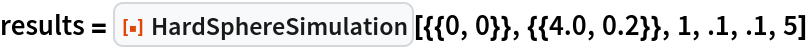Out=Plot the position at each time:

 In:=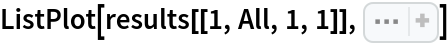Out=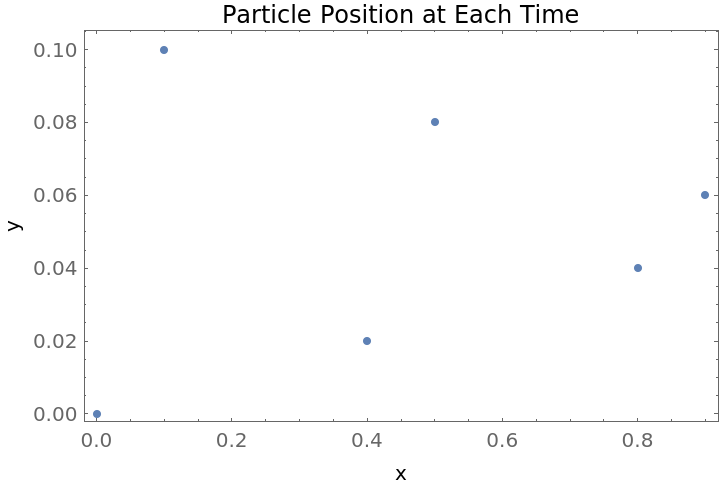### Scope (3)

Use HardSphereSimulation to visualize the evolution of a single hard sphere in a box:

 In:=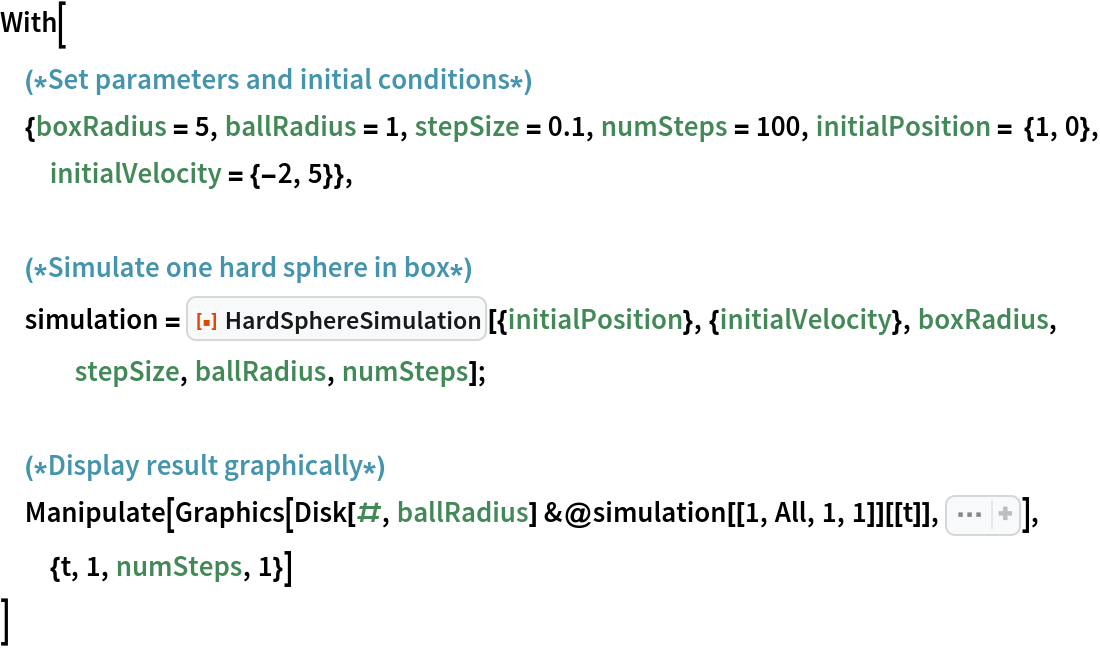Out=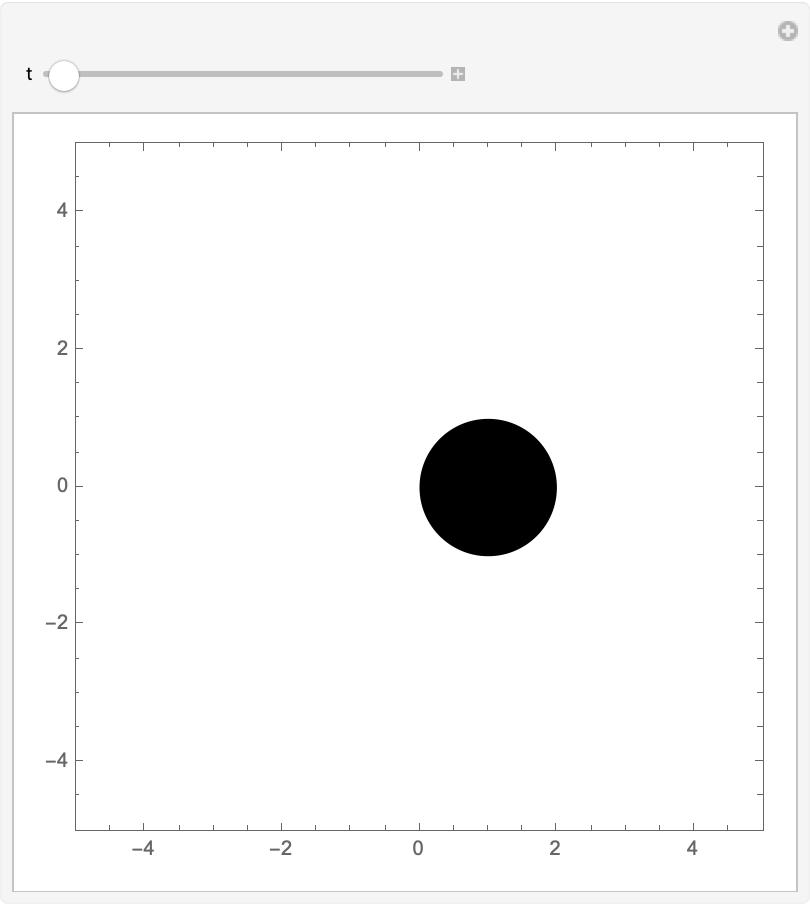Use HardSphereSimulation to visualize many hard spheres moving in a box:

 In:=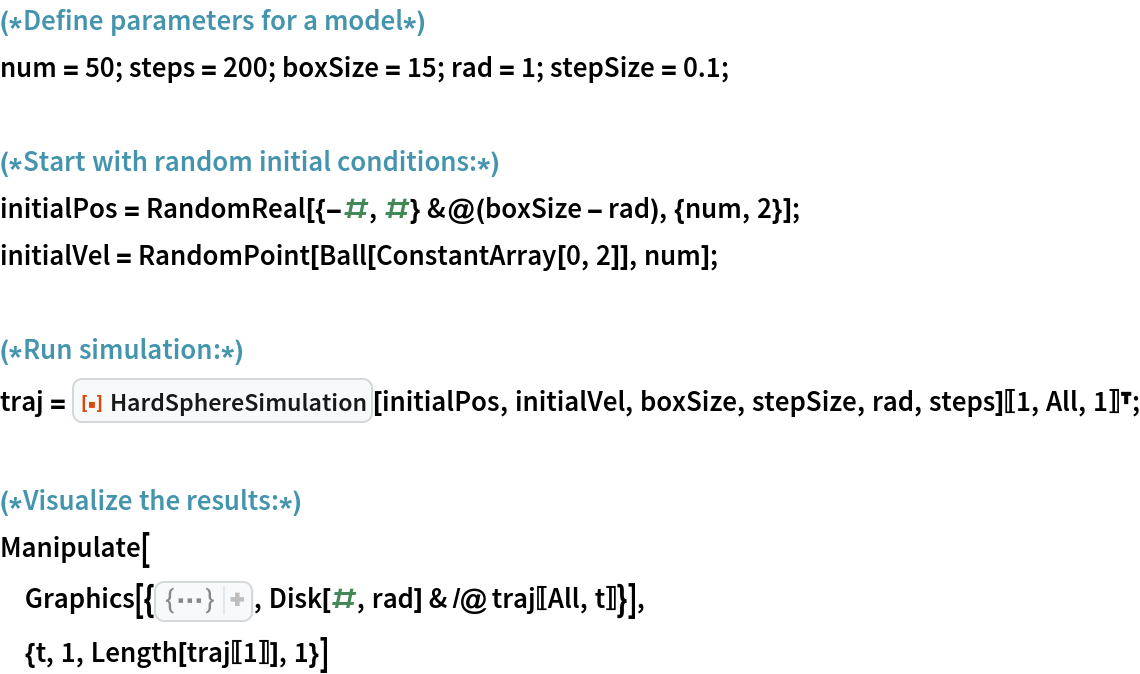Out=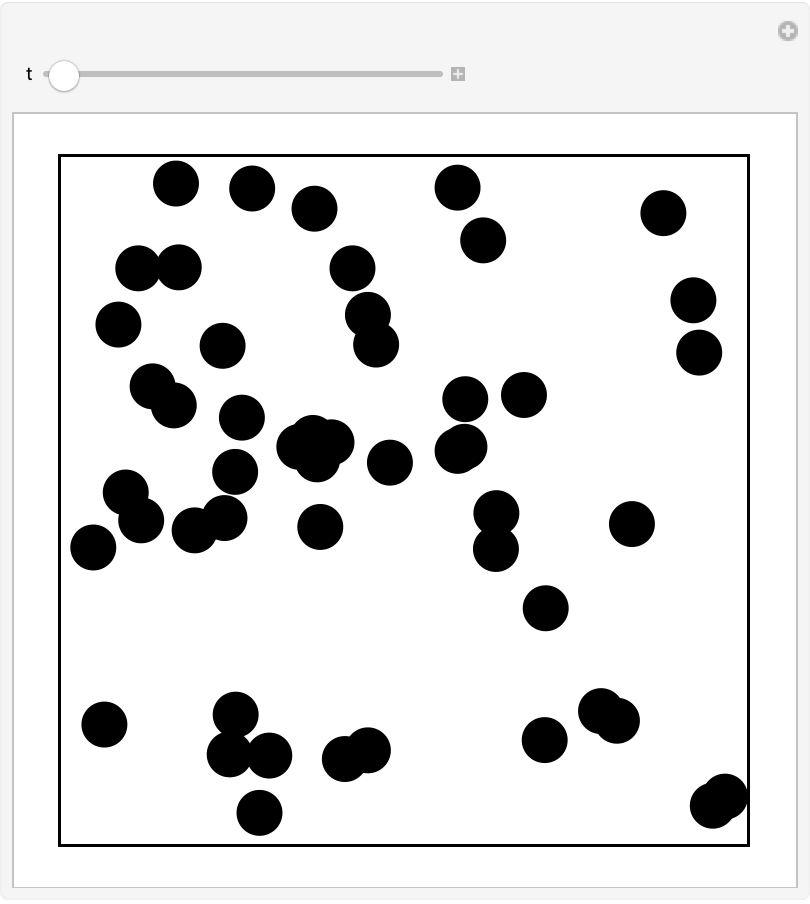Derive causal graph from list of collisions:

 In:=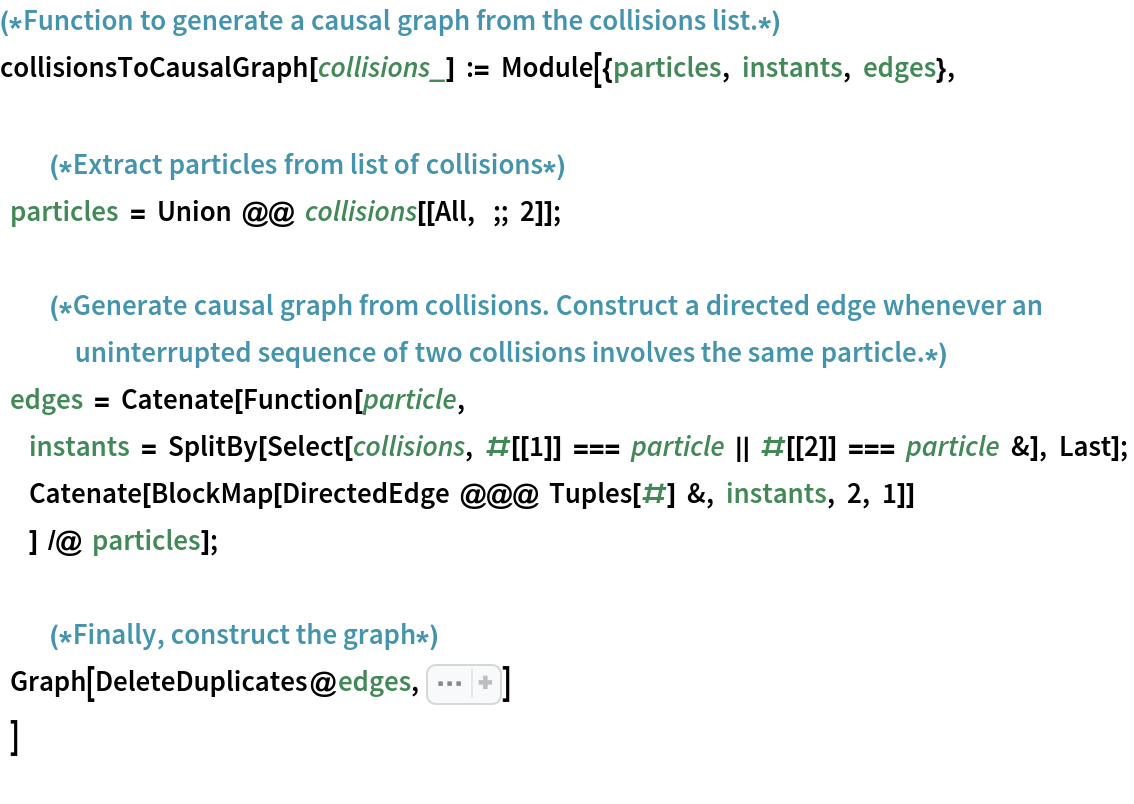In:=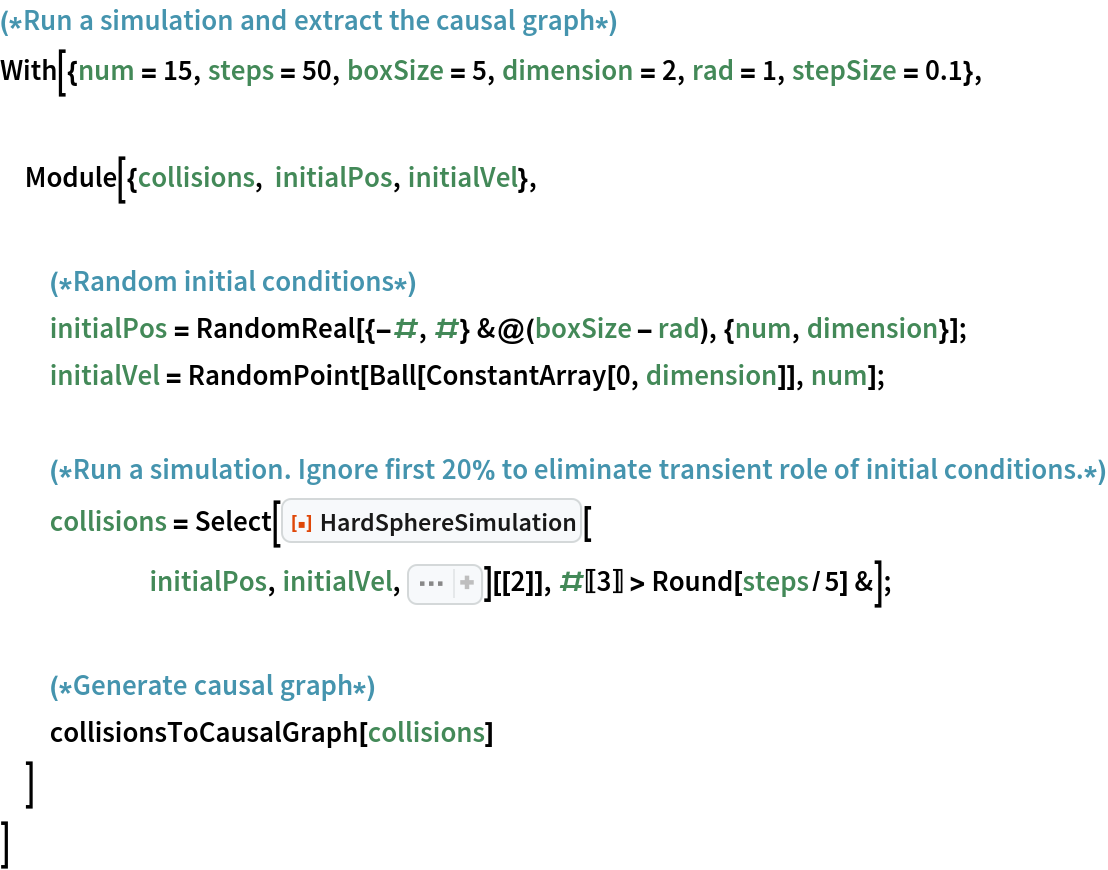Out=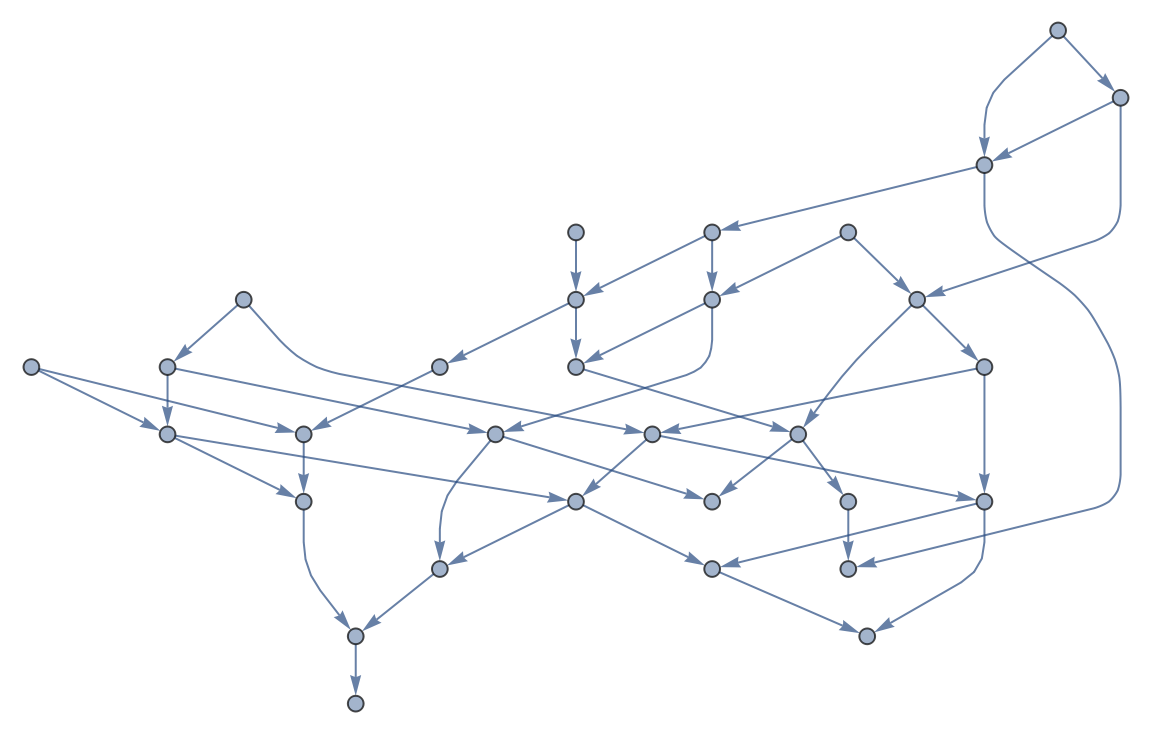### Properties and Relations (1)

Total kinetic energy of spheres should be conserved in time:

 In:=In:=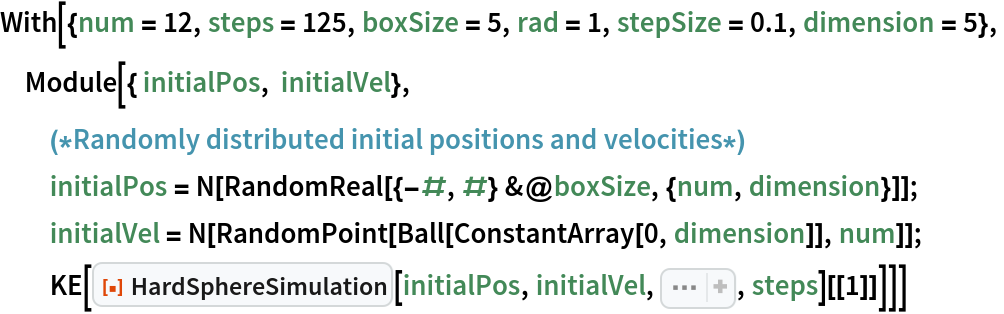Out=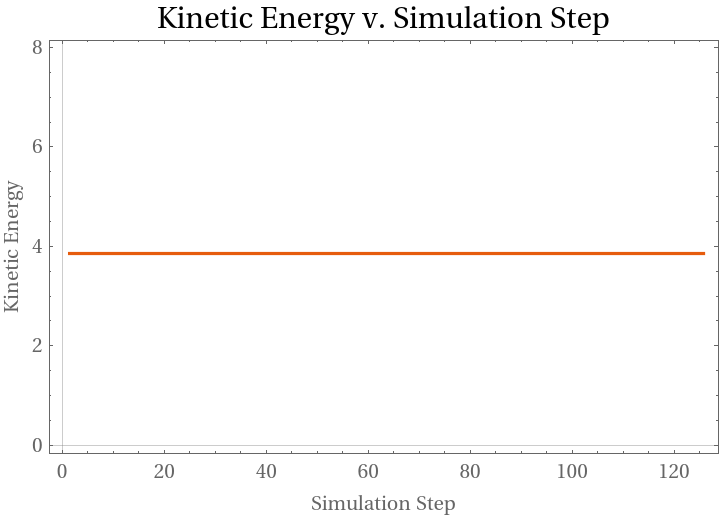### Neat Examples (1)

Use HardSphereSimulation to simulate simplified pool:

 In:=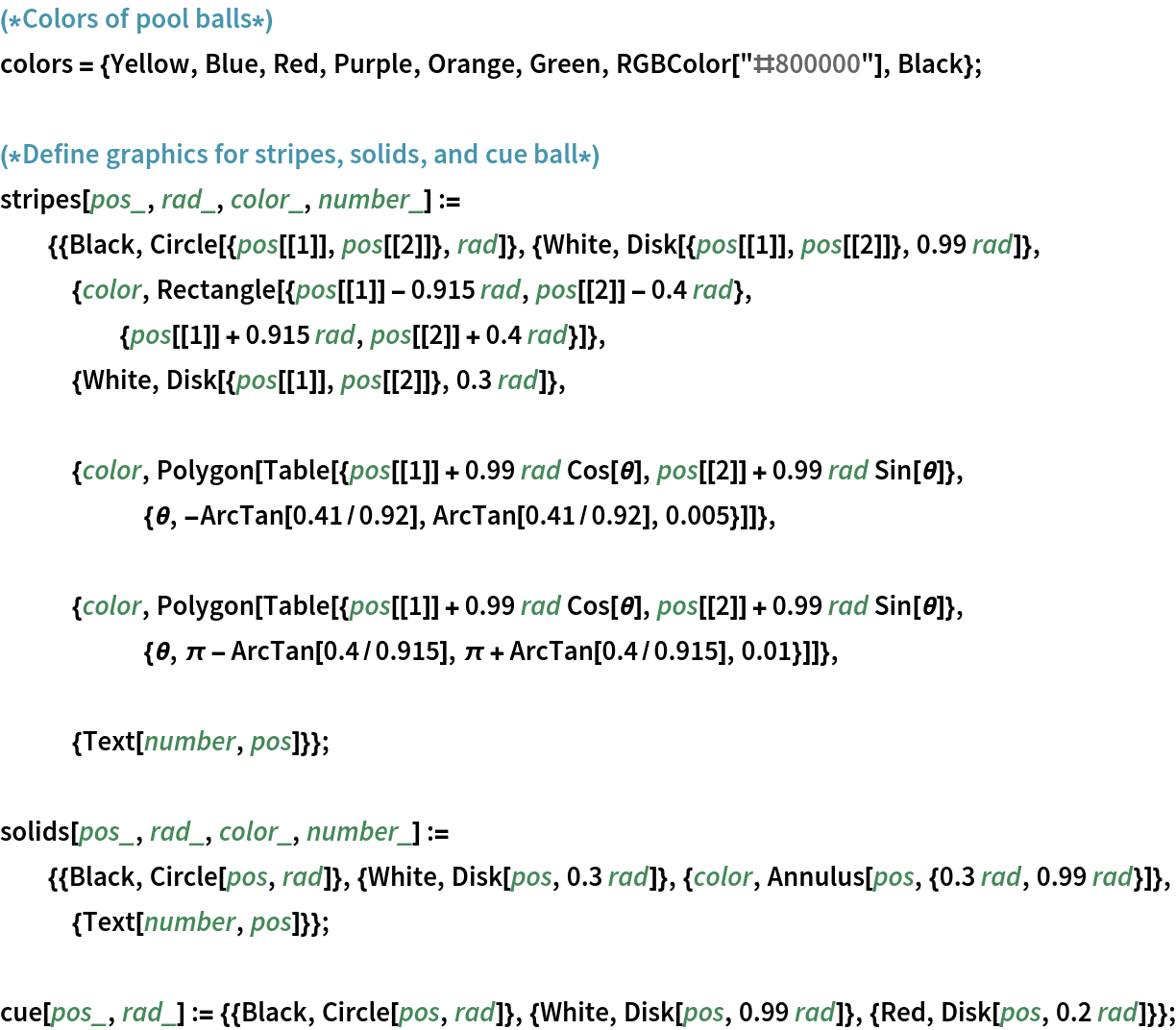In:=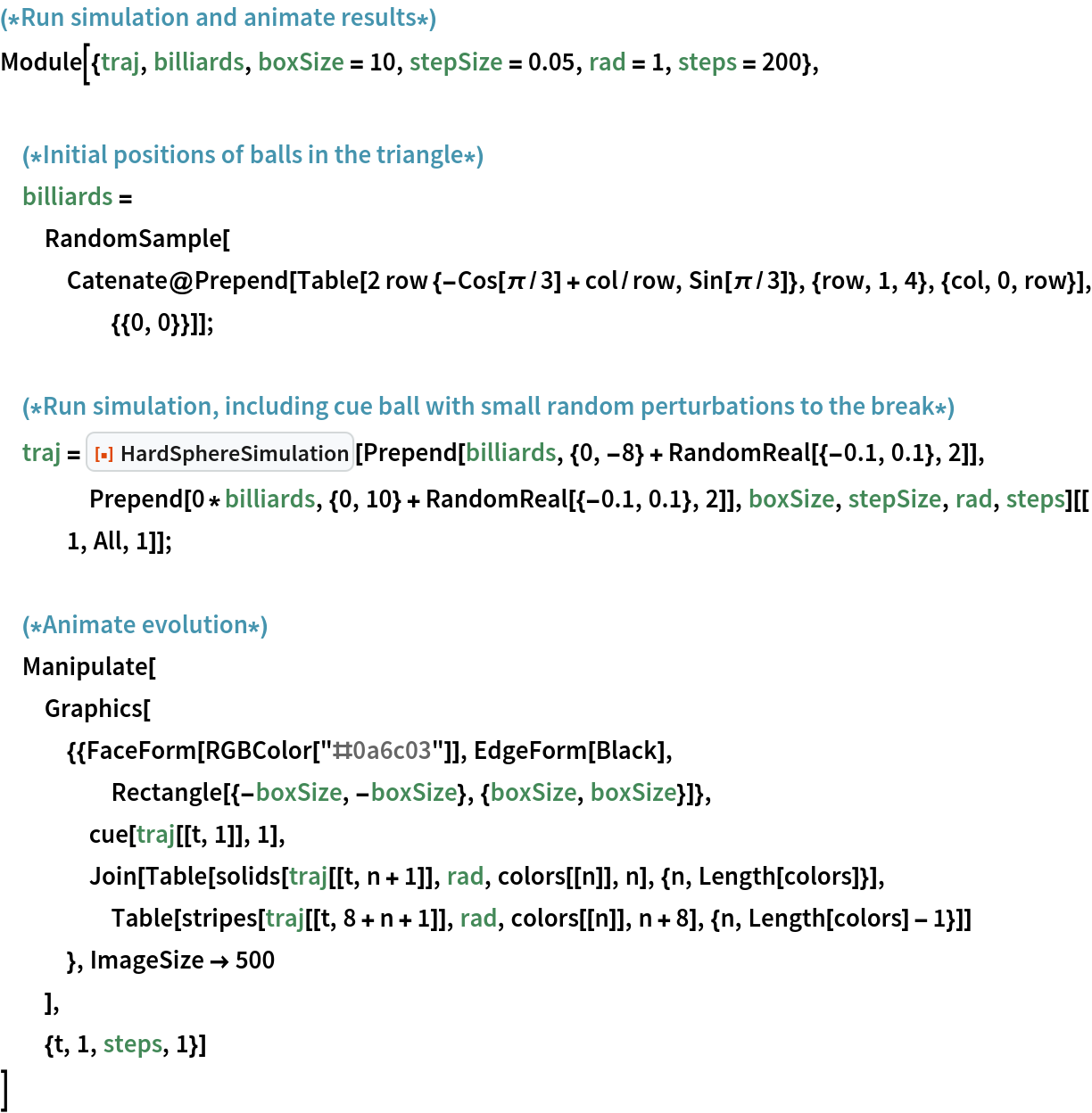Out=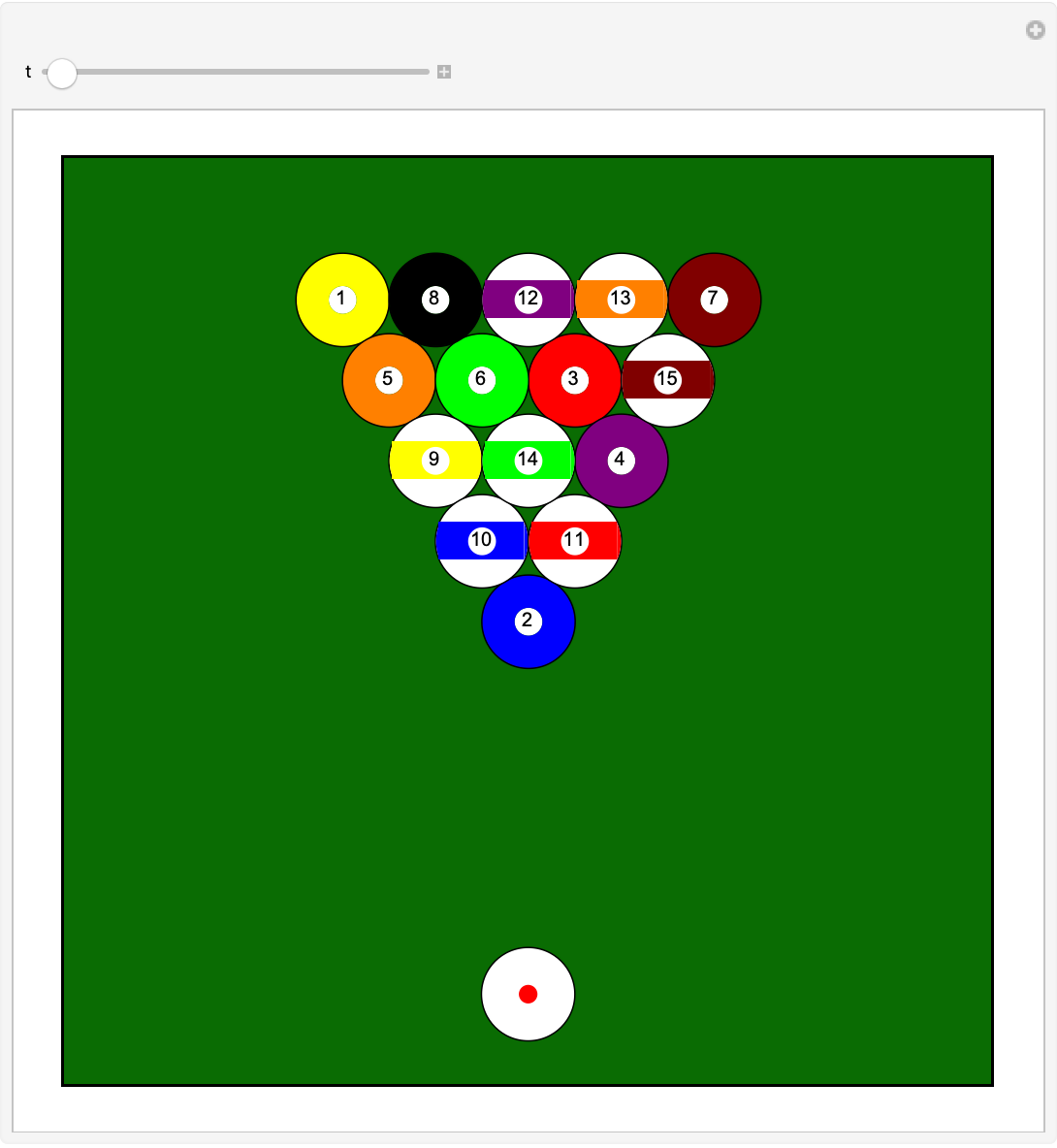## Publisher

Wolfram Summer School

## Version History

• 2.1.0 – 30 December 2022
• 2.0.1 – 14 October 2022
• 2.0.0 – 28 September 2022
• 1.0.0 – 16 February 2021